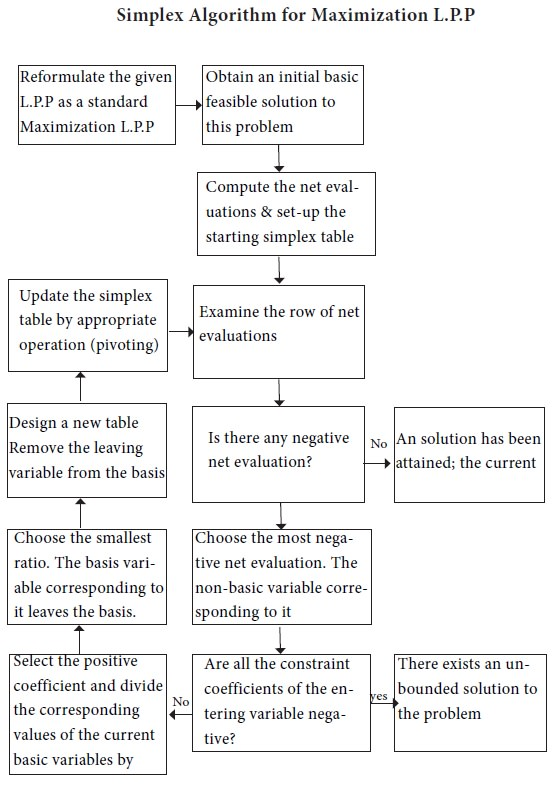Home | ARTS | Operations Management | Simplex Algorithm - Linear Programming – Problem Solving [SIMPLEX METHOD]

# Simplex Algorithm - Linear Programming – Problem Solving [SIMPLEX METHOD]

Posted On :  22.06.2018 10:43 pm

Check whether the objective function of the given L.P.P. is to be maximized or minimized.

Simplex Algorithm

## Step 1

Check whether the objective function of the given L.P.P. is to be maximized or minimized.

If it is to be minimized then convert it into a problem of maximizing it by using the result

Minimum= -Maximum (-z)

## Step 2

Check whether all ‘b’ values are non-negative. If any one of b is negative then multiply the corresponding inequality constraints by –1, so as to get all b values as non-negative.

## Step 3

Convert all the in equations of the constraints into equations by introducing slack and/or surplus variables in the constraints. Put the costs of these variables equal to zero in the objective function, if the variables are slack variables. If surplus / artificial variables are added, then we need to use ‘Big M’ Method, which is a modified algorithm of the same simplex method.

## Step 4

Obtain an initial basic feasible solution to the problem in the form Xb=B^-1 b and put it in the first column of the simplex table.

## Step 5

Compute the net evaluations zj-cj (j=1,2…n) by using the relation,

Zj-Cj=CB yj-cj.

Examine the sign zj-cj.

i.        If all values are >=0, then initial basic feasible solution is an optimum feasible solution.

ii.      If at least one value < 0, go to next step.

## Step 6

If there is more than one negative value, then choose most negative.

## Step 7

Compute the ratio

{xb/yi, yi>0,I=1,2…. m} and choose the minimum of them.

The common element in the kth row and rth column is known as the leading element (pivotal element) of the table.

## Step 8

Convert the leading element to unity by dividing its row by the leading element itself and all other elements in its column to zeros.

## Step 9

Go to step 5 and repeat the process until either an optimum solution is obtained or there is an indication of unbounded solution.Tags : Operations Management - Introduction to Operations Research
Last 30 days 1450 views